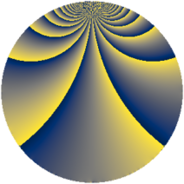# Properties

 Label 152.2.tLevel $152$ Weight $2$ Character orbit 152.t Rep. character $\chi_{152}(5,\cdot)$ Character field $\Q(\zeta_{18})$ Dimension $108$ Newform subspaces $1$ Sturm bound $40$ Trace bound $0$

# Related objects

## Defining parameters

 Level: $$N$$ $$=$$ $$152 = 2^{3} \cdot 19$$ Weight: $$k$$ $$=$$ $$2$$ Character orbit: $$[\chi]$$ $$=$$ 152.t (of order $$18$$ and degree $$6$$) Character conductor: $$\operatorname{cond}(\chi)$$ $$=$$ $$152$$ Character field: $$\Q(\zeta_{18})$$ Newform subspaces: $$1$$ Sturm bound: $$40$$ Trace bound: $$0$$

## Dimensions

The following table gives the dimensions of various subspaces of $$M_{2}(152, [\chi])$$.

Total New Old
Modular forms 132 132 0
Cusp forms 108 108 0
Eisenstein series 24 24 0

## Trace form

 $$108q - 6q^{2} - 12q^{4} - 12q^{6} - 6q^{7} - 3q^{8} - 12q^{9} + O(q^{10})$$ $$108q - 6q^{2} - 12q^{4} - 12q^{6} - 6q^{7} - 3q^{8} - 12q^{9} + 9q^{10} - 3q^{12} - 9q^{14} - 12q^{15} - 12q^{17} - 12q^{18} - 42q^{20} - 12q^{22} - 12q^{23} - 36q^{24} - 12q^{25} + 21q^{26} + 24q^{28} - 48q^{30} + 30q^{31} + 39q^{32} - 30q^{33} - 60q^{34} + 69q^{36} - 42q^{38} - 24q^{39} + 36q^{40} - 24q^{41} - 81q^{42} + 45q^{44} - 18q^{46} - 48q^{47} - 21q^{48} - 24q^{49} - 12q^{50} + 3q^{52} + 63q^{54} - 42q^{55} + 30q^{56} - 12q^{57} - 84q^{58} + 30q^{60} - 6q^{62} + 30q^{63} + 3q^{64} - 6q^{65} + 54q^{66} + 36q^{68} + 123q^{70} - 12q^{71} + 150q^{72} + 12q^{73} + 75q^{74} + 42q^{76} + 39q^{78} - 12q^{79} + 51q^{80} - 18q^{81} + 99q^{82} + 75q^{84} - 48q^{86} - 6q^{87} - 27q^{88} - 12q^{89} + 66q^{90} - 48q^{92} + 54q^{94} - 72q^{95} + 42q^{96} - 12q^{97} + 93q^{98} + O(q^{100})$$

## Decomposition of $$S_{2}^{\mathrm{new}}(152, [\chi])$$ into newform subspaces

Label Dim. $$A$$ Field CM Traces $q$-expansion
$$a_2$$ $$a_3$$ $$a_5$$ $$a_7$$
152.2.t.a $$108$$ $$1.214$$ None $$-6$$ $$0$$ $$0$$ $$-6$$